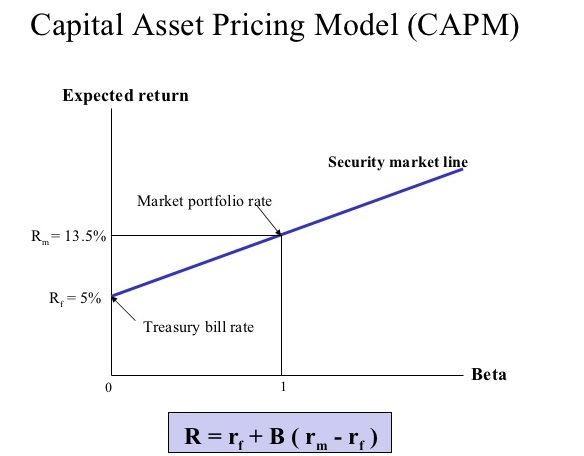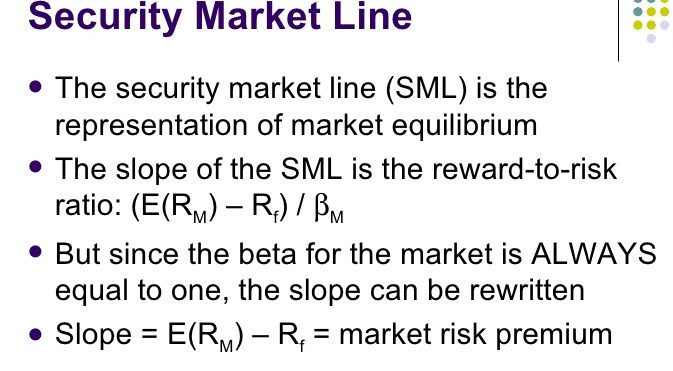Latest Articles
Home / Finance / Corporate Finance / How CAPM relates with the Risk and Return

# How CAPM relates with the Risk and Return

How CAPM relates to the risk and return.  The standard deviation of an individual stock does not indicate how that stock will contribute to the risk and return of a diversified portfolio. Thus, another measure of risk is needed; a measure of a security’s systematic risk. This measure is provided by the Capital Asset Pricing Model (CAPM).

The Capital Asset Pricing Model (CAPM) provides an expression that relates the expected return on an asset to its systematic risk. The relationship is known as the Security Market Line (SML) equation and the measure of systematic risk in the CAPM is called Beta.

Page Contents

# How CAPM relates to the risk and returnFigure – Capital Asset Pricing Model (CAPM)

## The Security Market Line (SML)

The security market line (SML) is a line that indicates a graphical demonstration of the capital asset pricing model (CAPM). The Security Market Line (SML) shows different levels of systematic, or market, risk of various marketable securities plotted against the expected return of the whole market at a specified point of time.

The Security Market Line (SML) also can be called as the “characteristic line”. Because it is a pictorial representation of CAPM (Capital Asset Pricing Model) –

## The y-axis of the chart represents the expected return.

The market risk premium of a given security is determined by where it is plotted on the chart in relation to the SML.

## The SML equation

The SML equation is expressed as follows-

where

E[Ri] = the expected return on asset i,
Rf = the risk-free rate,
E[Rm] = the expected return on the market portfolio,
bi = the Beta on asset i, and
E[Rm] – Rf = the market risk
The graph below depicts the SML. Note that the slope of the SML is equal to (E[Rm] – Rf) which is the market risk premium and that the SML intercepts the y-axis at the risk-free rate.In capital market equilibrium, the required return on an asset must equal its expected return.

How CAPM relates to the Risk and Return